### Number Bases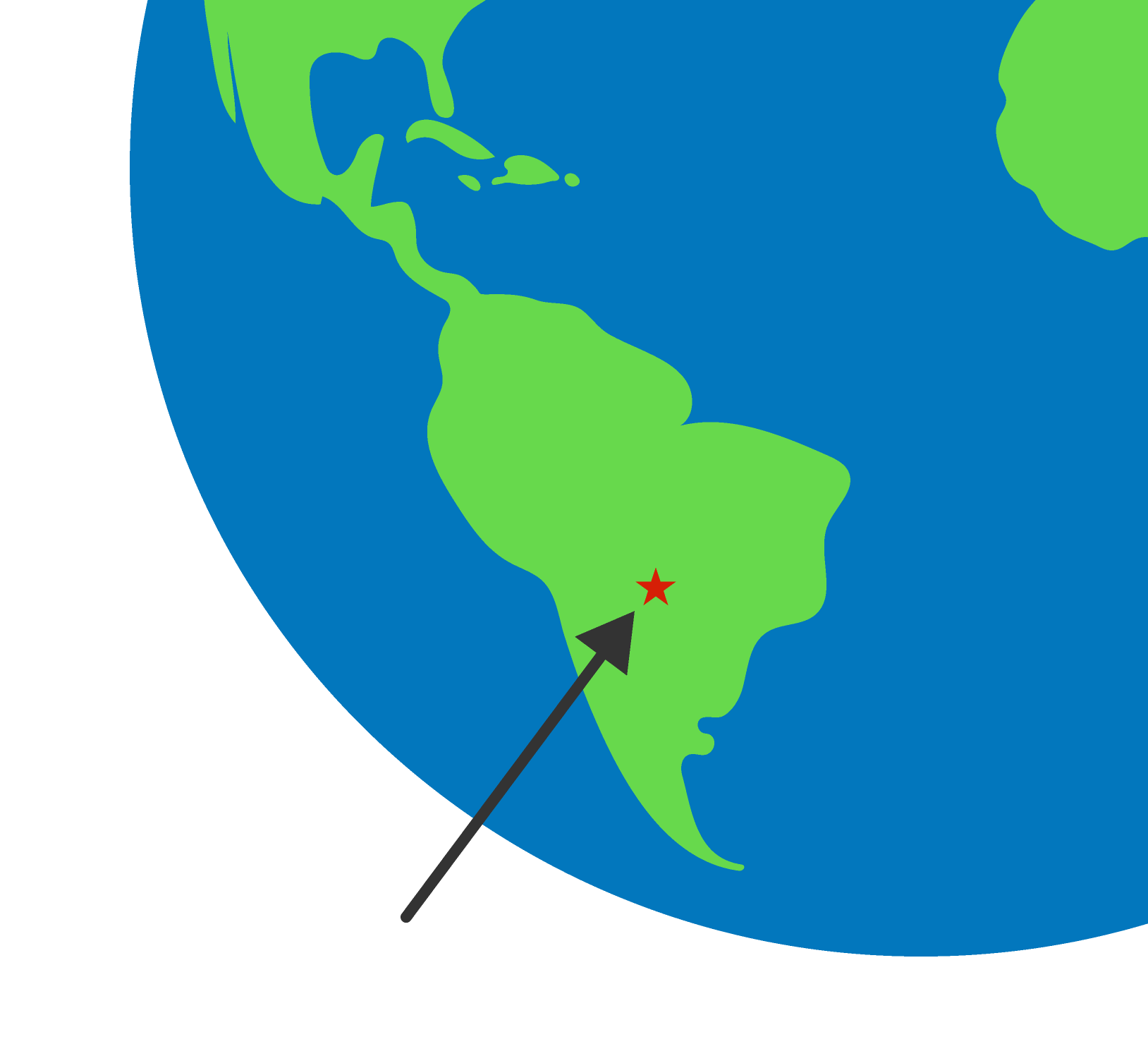In their native language, the Bakairi of South America have an additive system for representing numbers:

1 = tokale
2 = azage
3 = azage tokale
4 = azage azage
5 = azage azage tokale
6 = azage azage azage

What number corresponds to "azage azage azage azage tokale"?

# The Invention of Number Bases

Using the same Bakairi style of number (tokale = 1, azage = 2, and tokale is used at most once), how many words would be needed to express the number 240?

# The Invention of Number Bases

It's fairly clear that

azage azage azage azage azage azage azage azage azage azage azage azage azage azage azage azage azage azage azage azage azage azage azage azage azage azage azage azage azage azage azage azage azage azage azage azage azage azage azage azage azage azage azage azage azage azage azage azage azage azage azage azage azage azage azage azage azage azage azage azage azage azage azage azage azage azage azage azage azage azage azage azage azage azage azage azage azage azage azage azage azage azage azage azage azage azage azage azage azage azage azage azage azage azage azage azage azage azage azage azage azage azage azage azage azage azage azage azage azage azage azage azage azage azage azage azage azage azage azage azage

is an impractical word to use in daily life. (The Bakairi now use Portuguese for numbers larger than two.)

This is a "counting" system, so the number words are just being added. The Bakairi only have words for one and two; what if we applied a counting system but used more number words?

# The Invention of Number Bases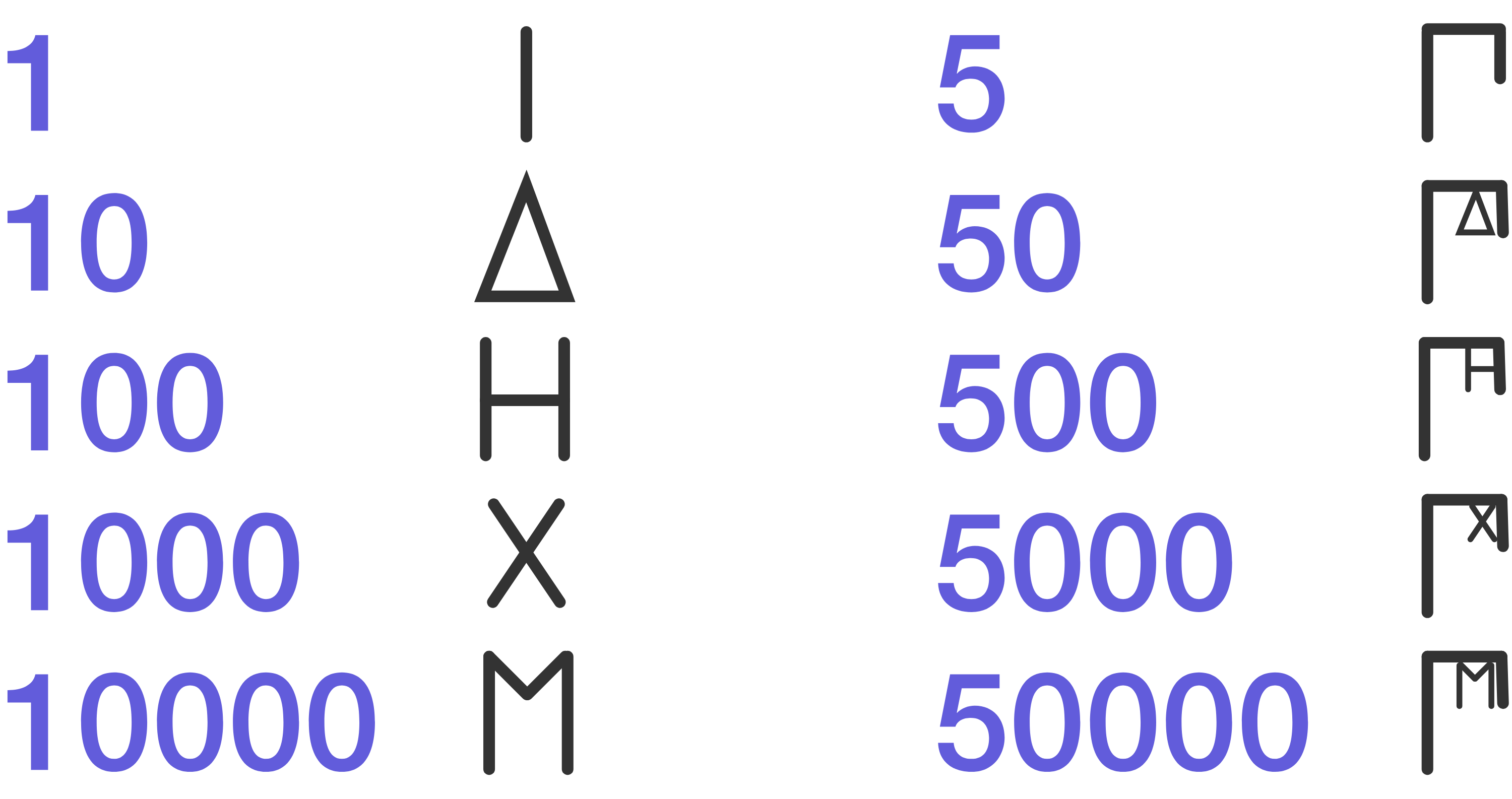The acrophonic system was used in ancient Greece for numbers, and it is additive as well.

Representing 632 in the acrophonic system uses seven symbols:$500 + 100 + 10 + 10 + 10 + 1 + 1 = 632.$

How many symbols are needed in the acrophonic system to represent 494?

# The Invention of Number Bases

Additive number systems result in unwieldy long numbers even when we add more words or symbols. A positional number system helps to alleviate this problem. Our modern number system is positional and called base-10 because it uses 10 digits (0 through 9).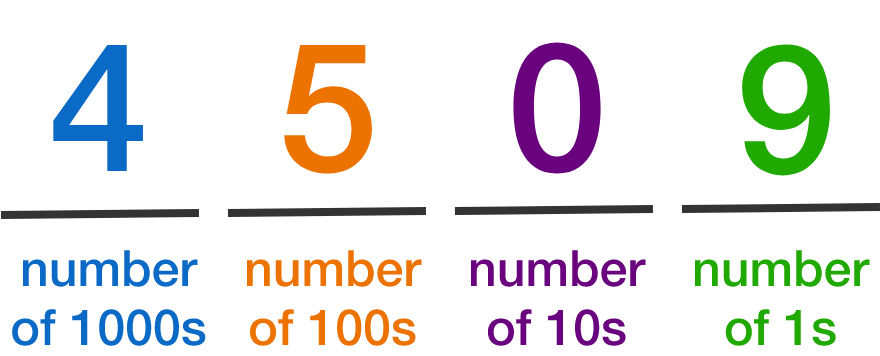The idea is to start counting from 0, and after reaching the last digit (9) the next number in sequence will "overflow":

1, 2, 3, 4, 5, 6, 7, 8, 9, 10

That is, in the number after 9 we have 10 ones, but we can't write that as a digit. So, we add a "tens" place and then say we have counted a single ten. Then we can reset the ones place back to 0.

Suppose instead of base 10 we used base 8, that is, an octal system with the digits 0 to 7. What goes at the question mark on the chart below? (Hint: this will be the same as the number 100 in octal converted to base 10.)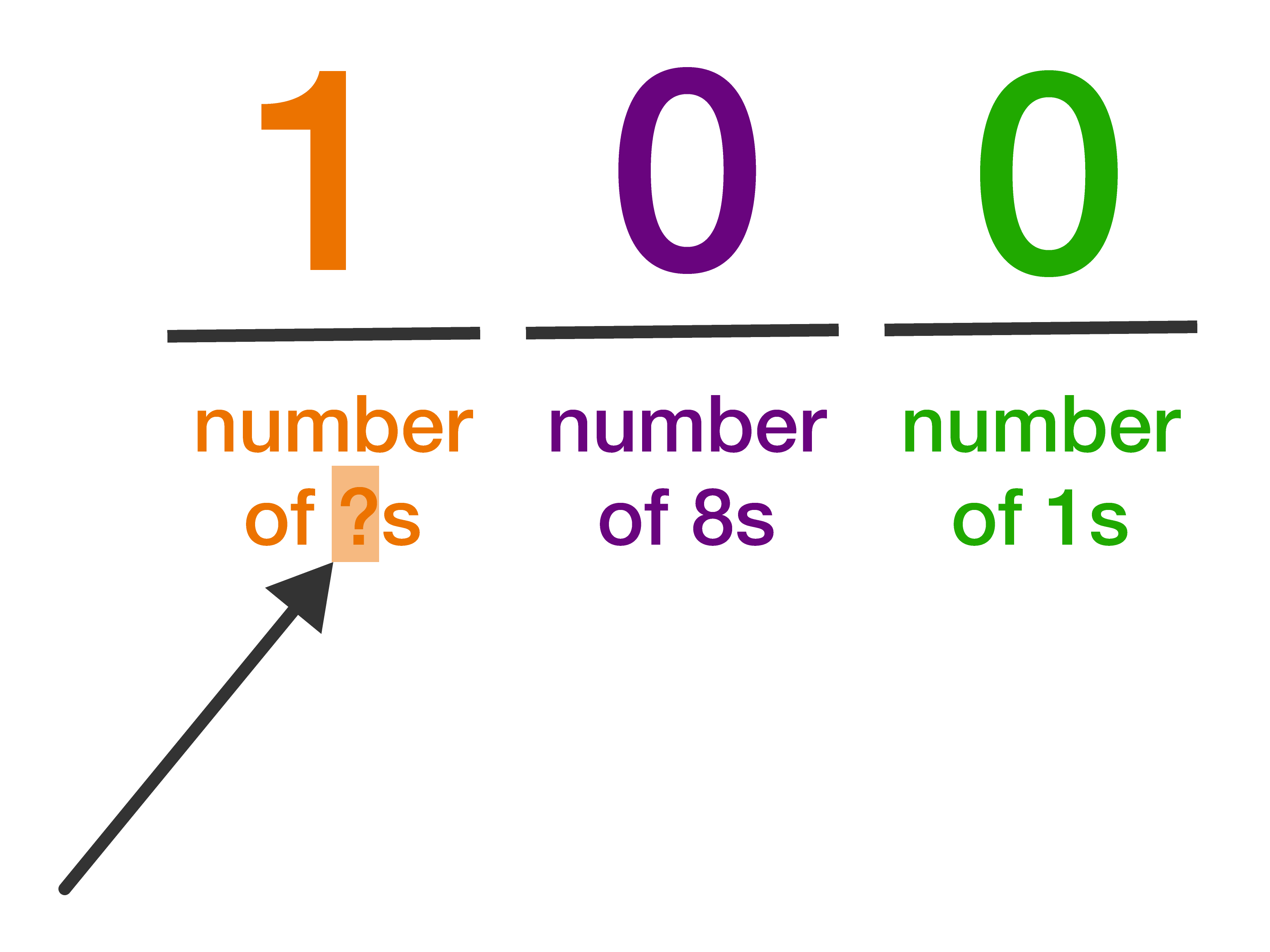# The Invention of Number Bases

Suppose we had a quinary number system where the only digits we could use were 0, 1, 2, 3, and 4. What's the largest number we could write with two digits (convert your result to base 10 before answering)?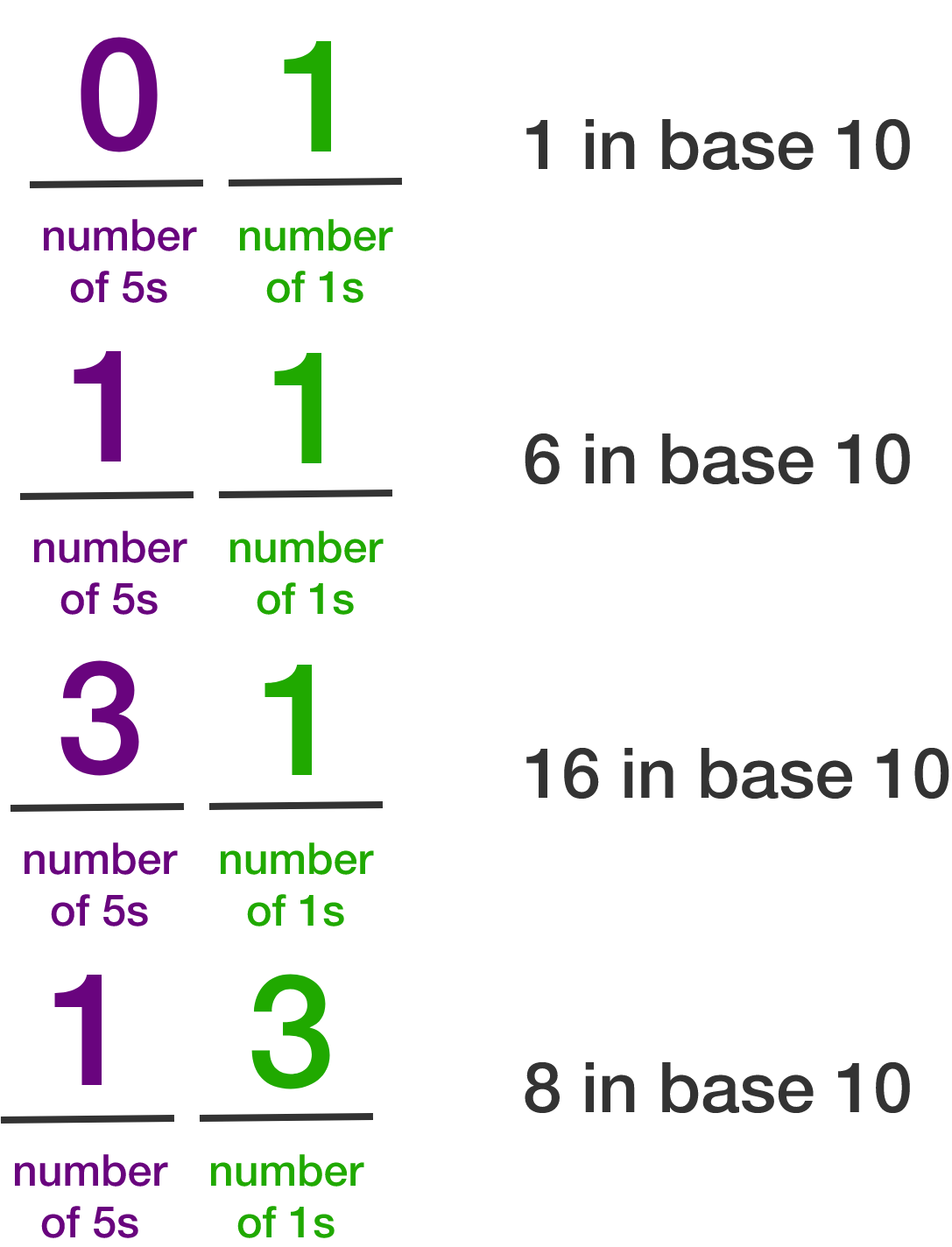# The Invention of Number Bases

It may initially seem esoteric to work in any system other than base 10, but for technical reasons it is very convenient for computers to work in base 2, also known as binary, only using the digits 0 and 1.For the rest of this introduction, we'll try some problems in base-2 and then finish by seeing specifically how it applies to computers (and how it manifests itself in unexpected ways).

×